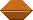The Bead Unbaffled - An Abacus Manual

###DECIMALS & MULTIPLICATION (contributed by Edvaldo Siqueira)

The following is a method used by Professor Fukutaro Kato, a Japanese soroban teacher living in Brazil in the 1960's. The method was published in the Professor's book, *SOROBAN pelo Método Moderno* which, when translated from Portuguese means *SOROBAN by the Modern Method*

This is an explanation on how to apply "Professor Kato's Method" for placing correctly the *multiplicand* on the soroban,in order that the unit digit of the *product* will fall always on a previously chosen unit rod. Bear in mind that the arithmetic operation should be carried out according Kojima's standard method for multiplication.

For the sake of clarity, let's split the following in 4 short sections: A) HOW TO COUNT DIGITS;  B) RULE TO FOLLOW;  C) NUMBERS ON THE SOROBAN;  and  D) A FEW EXAMPLES.

A) COUNTING DIGITS

i) If the *multiplicand or the multiplier* are integers or mixed numbers (those with a whole part and decimal places), consider in each one only the digits in their whole part. So disregard any decimal digit, after the point, if any.

Ex:

```3.105......has 1 digit;
47.02......has 2 digits
308.41.....has 3 digits
1000.......has 4 digits and so on```

ii) When the number is a pure decimal, consider (count) only those trailing zeros (if any) before the first significant digit attributing to the total a negative sign. Disregard the zero before the point

Ex:

```0.00084.....has -3 digits
0.40087.....has  0 digits
0.01010.....has -1 digit```

B) RULES TO FOLLOW

B1) Compute the Number: N = # digits on multiplicand + # digits on multiplier + 1

Note: Before computing N, please note that number 1 should always be added to the # of digits on the multiplier.

B2) EXAMPLES:

```30 x 8.............N = 2 + 1 + 1 = 4
2 x 3.14...........N = 1 + 1 + 1 = 3
12 x 0.75..........N = 2 + 0 + 1 = 3
0.97 x 0.1.........N = 0 + 0 + 1 = 1
0.5 x 8.14.........N = 0 + 1 + 1 = 2
0.03 x 0.001.......N = -1 + (-2) + 1 = -2```

C) NUMBERS ON THE SOROBAN

```<----A----B----C----D----E---*F*---G----H----I----J-->
<...+6...+5...+4...+3...+2...+1....0...-1...-2...-3..>```

Let's associate an orientating axis extending from right to left of the soroban rods, with the axis positive number 1 corresponding to the *unit rod* (*F*). That correspondence is paramount to the correct application of this method! Obviously, the rod chosen to be carry the unit can be anyone - towards the center of the soroban - provided it is marked with a dot. To the left of *F* (rods E, D, C ...) we have positive values; to its right, Zero and negative values, as shown. Imagine the above diagram extending to both sides as far as necessary to work comfortably according Kojima's. You will soon note that when the absolute value of N "large" (say, > 4), the other printed dots on the bar are handy in choosing the appropriate rod for setting the multiplicand highest order digit.

D) EXAMPLES

i) 40.0 x 0.225 = 9,
N = 2 + 0 + 1 = 3   ----> set 4 on D...product 9 will fall on *F*

ii) 75 x 12 = 900
N = 2 + 2 + 1 = 5   ----> set 75 on BC... After the multiplication process is carried out, you'll be left with only a 9 placed on rod D; but as *F* carries always the unit result digit, the product must be automatically read: 900.

iii) 0.400 x 0.025 = 0.010
N = 0 + (-1) +1 = 0   ----> set 4 on G...(From the product = 0.01, you only see of course the number 1 on rod H, which should be read as 0.01 - because 0 ( = the unit), is as always located on *F*.

iv) 2.6 x 3.5 = 9.1
N = 1 + 1 + 1 =3   ----> set 26 on DE...(product 91 will fall on FG; read as 9.1).

With practice, one will be able to compute N mentally and soon you should be using this alternative method correctly and confidently. The bonus is at the same time you will learn how to multiply decimal numbers on the soroban.

Quiz: Try to do the above Examples on B2, choose the soroban appropriate rod to place the highest order digit of the multiplicand; do again each exercise after computing mentally its expression for N; eventually do on your soroban each multiplication suggested.

Good luck!

Edvaldo Siqueira
Rio de Janeiro, Brazil